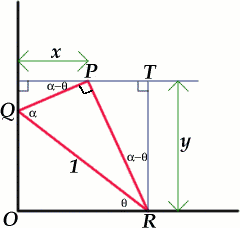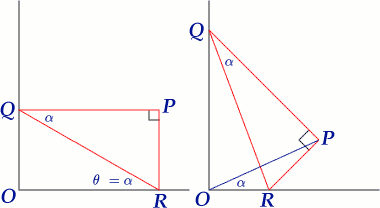#### You may also like### Five Circuits, Seven Spins

A circular plate rolls inside a rectangular tray making five circuits and rotating about its centre seven times. Find the dimensions of the tray.### Mach Attack

Have you got the Mach knack? Discover the mathematics behind exceeding the sound barrier.### Construct the Solar System

Make an accurate diagram of the solar system and explore the concept of a grand conjunction.

# Set Square

##### Age 16 to 18Challenge Level

The locus of the point $P$ is a straight line as the vertices $Q$ and $R$ slide along the walls.Sue Liu of Madras College, St Andrew's sent this solution to the problem. Without loss of generality we can let the length of $QR$ be 1 unit, and take a coordinate system with the origin at $O$ and axes along $OR$ and $OQ$. If $\angle PQR = \alpha$, where $0 < \alpha < 90^\circ$, then $PQ = \cos \alpha$ and $PR = \sin \alpha.$ Let $\angle QRO = \theta$ where $0 \leq \theta \leq 90^\circ$. Then, from the right angled triangles $PSQ$ and $PTR$, we have $\angle PRT = \angle QPS = \alpha - \theta$, and hence we can write down the coordinates of the point $P$.

\begin{eqnarray} x &=& \cos \alpha \cos (\alpha - \theta) \\ y &=& \sin \alpha \cos (\alpha - \theta). \end{eqnarray}
We see that $${y\over x}= {\sin \alpha \cos (\alpha - \theta)\over \cos \alpha \cos (\alpha - \theta)}= \tan \alpha.$$ and so $P$ lies on the straight line $y = x\tan \alpha$.

The position $(x,y)$ depends only on $cos(\alpha - \theta)$, $\alpha$ being a constant, and $\theta$ a variable. The distance of the point $P$ from $O$ is given by $$OP^2 = x^2 + y^2 = \cos^2(\alpha - \theta)(\cos^2\alpha + \sin^2\alpha) = \cos^2(\alpha - \theta).$$ Hence $OP = \cos(\alpha - \theta)$ which is a maximum when $\cos(\alpha - \theta) = 1$, that is when $\alpha = \theta$. This occurs when $OQPR$ is a rectangle as shown in the diagram.We get an even simpler method of solution by using the fact that the angles $QOR$ and $QPR$ are both 90 degrees so that $OQPR$ is a cyclic quadrilateral with $PR$ as a chord. We have $\angle POR = \angle PQR = \alpha$ because these two angles are subtended by the same chord of the circle. This shows that $\angle POR$ is constant and hence that the locus of $P$ is the straight line $y = x \tan \alpha.$

What can you say about the locus of $P$ if the triangle $PQR$ is not a right angled triangle?